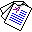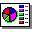Equivalence of Logic Programs under Updates

# Equivalence of Logic Programs under Updates

## Katsumi Inoue and Chiaki Sakama

Proceedings of the 9th European Conference on Logics in Artificial Intelligence (JELIA'04), Lecture Notes in Artificial Intelligence 3229, Springer-Verlag, pages 174-186, 2004.

## Abstract

This paper defines a general framework for testing equivalence of logic programs with respect to two parameters. Given two sets of rules {\cal Q} and {\cal R}, two logic programs P1 and P2 are said to be update equivalent with respect to ({\cal Q},{\cal R}) if (P1\Q)\cup R and (P2\Q)\cup R have the same answer sets for any two logic programs Q\subseteq{\cal Q} and R\subseteq{\cal R}. The notion of update equivalence is suitable to take program updates into account when two logic programs are compared. That is, the notion of relativity stipulates the languages of updates, and two parameters {\cal Q} and {\cal R} correspond to the languages for deletion and addition, respectively. Clearly, the notion of strong equivalence is a special case of update equivalence where {\cal Q} is empty and {\cal R} is the set of all rules in the language. In fact, the notion of update equivalence is strong enough to capture many other notions such as weak equivalence, update equivalence on common rules, and uniform equivalence. We also discuss computation and complexity of update equivalence.

Keywords: update equivalence, strong equivalence, computational complexityFull Paper (pdf 143K)Slide (pdf 129K) © Springer-Verlag